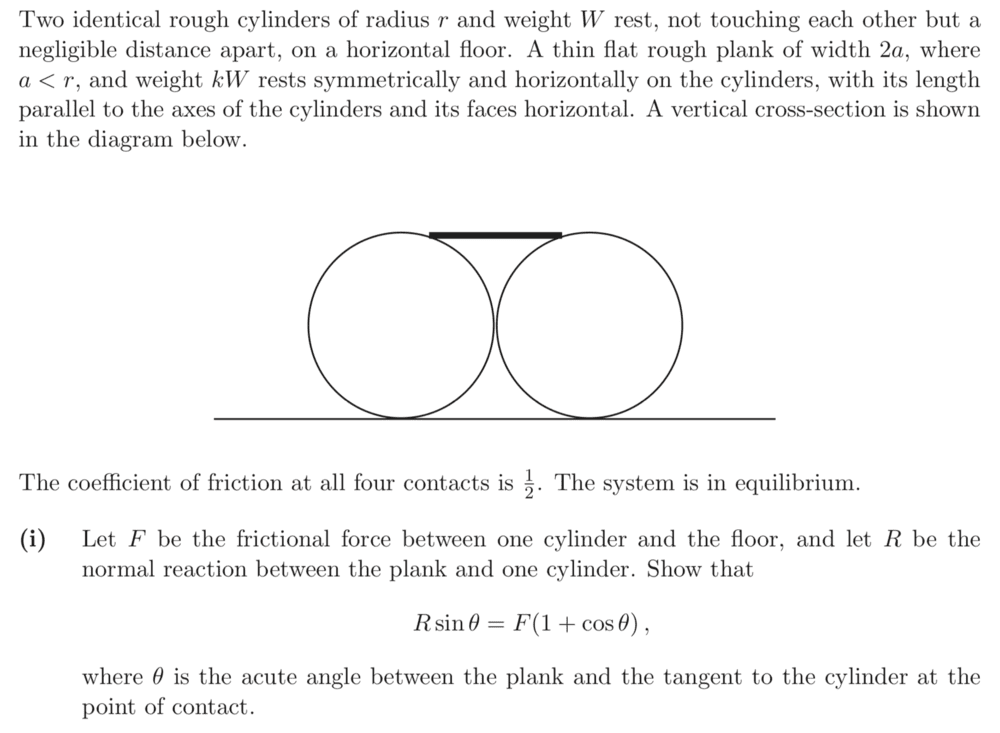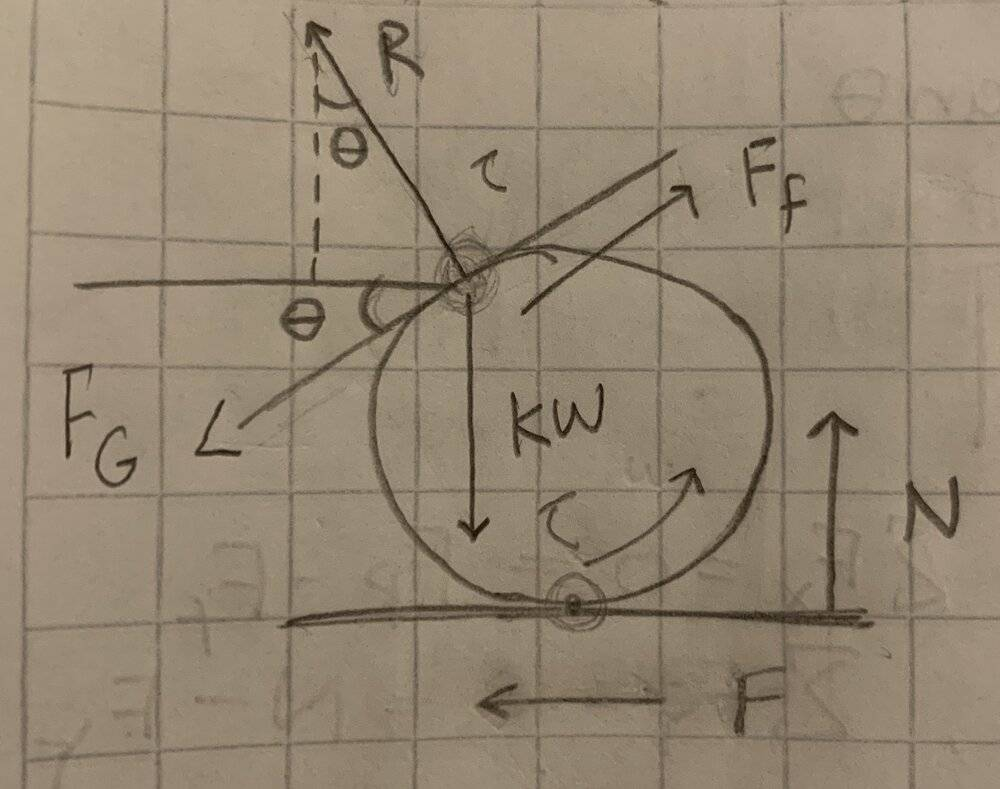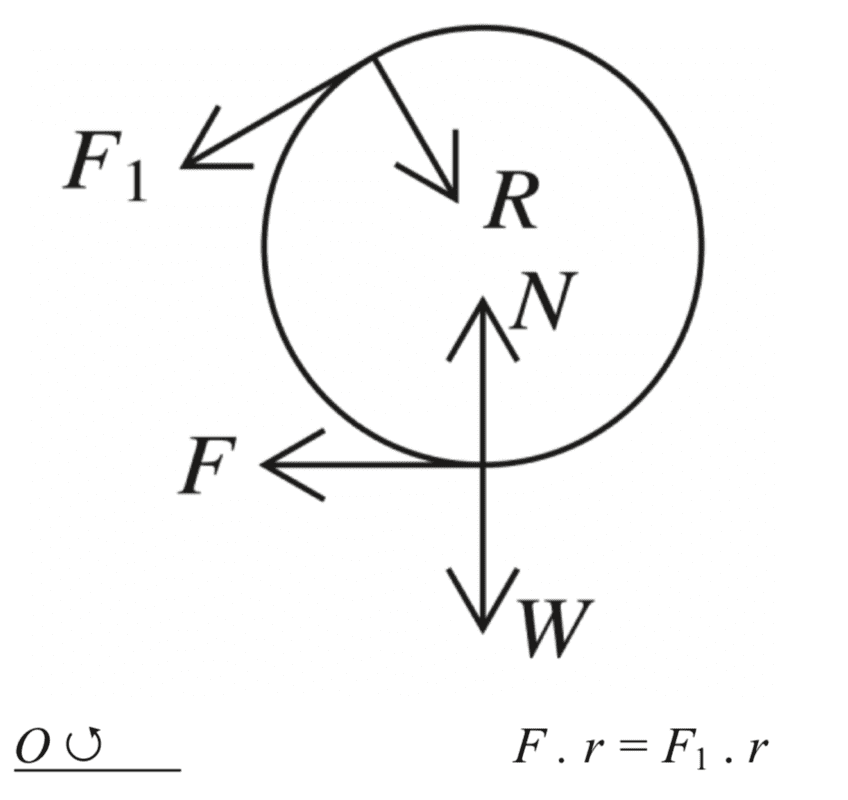# Free body Diagram of a Plank resting on two Cylinders

• Stewie117
In summary, the conversation discusses the problem of determining the direction of the frictional force F1 between a plank and a cylinder, as well as the normal force R and the force F acting on the cylinder. The solution involves drawing a free body diagram of the cylinder and balancing torques about the point of contact with the ground. It is determined that F1 must point in the opposite direction of R, and F must point left to counter the torque created by F1. f

#### Stewie117

Homework Statement
Two identical rough cylinders of radius r and weight W rest, not touching each other but a negligible distance apart, on a horizontal floor. A thin flat rough plank of width 2a, where a < r, and weight kW rests symmetrically and horizontally on the cylinders, with its length parallel to the axes of the cylinders and its faces horizontal. A vertical cross-section is shown in the diagram below.
The coefficient of friction at all four contacts is 1/2. The system is in equilibrium. 2
(i) Let F be the frictional force between one cylinder and the floor, and let R be the normal reaction between the plank and one cylinder. Draw a free body diagram and show that
Fsin(theta) = R(1+cos(theta))
Relevant Equations
Net torque = 0, Net force in horizontal direction = 0.I have put a picture of the problem above. The picture below shows my free body diagram. Fg is the component of the gravitational force in the direction fo the tangent at the point of contact and Ff is the frictional force between the plank and cylinder. I put Ff in the opposite direction as Fg since the plank naturally wants to slide downwards.The answer has this free body diagram:I understand the equations (you got to use the fact that the net torque and net force in the horizontal direction is zero), but I do not know why in the answer diagram F1 (which is the frictional force between the plank and cylinder) is what causes the anti clockwise rotation instead of Fg (which in my diagram represent the component of the gravitational force on the plank which is in the direction of the tangent at the point of contact). Also, I don't understand how the direction of R was chosen in the answer diagram. If somebody can help, pose do so. Thanks.

#### Attachments

Hello @Stewie , ##\qquad####\qquad## !

since the plank naturally wants to slide downwards
Sure, but you were supposed to make a free body diagram of the cylinder !
(at least, that's what the book solution does --- and it's useful for the torque balance))
So with the forces acting on the cylinder.

The force ##kW## does not act on the cylinder, it acts on the plank. The only forces at the interface between the plank and the cylinder are a normal reaction force and a frictional force. Taking torques about the point of contact with the ground once you have drawn your free body diagram for the cylinder should allow you to determine the direction of the frictional force.

Hello @Stewie , ##\qquad####\qquad## !

Sure, but you were supposed to make a free body diagram of the cylinder !
(at least, that's what the book solution does --- and it's useful for the torque balance))
So with the forces acting on the cylinder.

Ok, so drawing the free body diagram of the cylinder means that I should draw R going into the cylinder (as it appears in the answer) since that's the normal force acting on the cylinder. Then, since the normal force R pushes into the cylinder (its x component pushes to the right), the frictional force F1 (its x component) must point the other way. Is this the right way of thinking about it?

Ok, so drawing the free body diagram of the cylinder means that I should draw R going into the cylinder (as it appears in the answer) since that's the normal force acting on the cylinder. Then, since the normal force R pushes into the cylinder (its x component pushes to the right), the frictional force F1 (its x component) must point the other way. Is this the right way of thinking about it?

I don't think that's sufficient, since you don't yet know how it compares to the other horizontal frictional force. Better to balance torques about the point of contact with the ground.

I don't think that's sufficient, since you don't yet know how it compares to the other horizontal frictional force. Better to balance torques about the point of contact with the ground.

So, I don't understand which way the friction between the cylinder and floor F should point. Can you explain how you would draw the free body diagram if you saw this problem? Thank you

So, I don't understand which way the friction between the cylinder and floor F should point. Can you explain how you would draw the free body diagram if you saw this problem? Thank you

Exactly as the one in the given answer. The same way you said it should point in #4, just not for the reason you stated.

Exactly as the one in the given answer. The same way you said it should point in #4, just not for the reason you stated.

Then what's the reason?? I understand why F1 and R point in their respective directions but why does F have to point left? R doesn't create a torque right? So, F has to counter the torque created by F1 and point left. Is this thinking correct?

R doesn't create a torque right?

It doesn't product a torque about the centre of mass, no, but I was talking about the point of contact with the ground (about which both the reaction force and frictional force at the cylinder-plank interface have a non-zero torque).

My point was that the torque of ##R## is clockwise about the point of contact with the ground, so the torque of ##F_1## need be anticlockwise. Hence why it points in the leftward direction.

It doesn't product a torque about the centre of mass, no, but I was talking about the point of contact with the ground (about which both the reaction force and frictional force at the cylinder-plank interface have a non-zero torque).

My point was that the torque of ##R## is clockwise about the point of contact with the ground, so the torque of ##F_1## need be anticlockwise. Hence why it points in the leftward direction.

Yes, but you can also look at the torques about the center of mass of the cylinder. In which case, F needs to point leftward to counter the torque of F1. Right?

Yes, but you can also look at the torques about the center of mass of the cylinder. In which case, F needs to point leftward to counter the torque of F1. Right?

Right, that's another valid way of looking at it. But that's not what you originally said:
Then, since the normal force R pushes into the cylinder (its x component pushes to the right), the frictional force F1 (its x component) must point the other way. Is this the right way of thinking about it?

In any case, this is a digression. Have you been able to make any progress on the problem?

Right, that's another valid way of looking at it. But that's not what you originally said:

In any case, this is a digression. Have you been able to make any progress on the problem?
Yeah I mostly get it now. One last thing: So, for this problem, its best to determine the directions of R and F1 by looking at the torques about the point of contact at the ground and then find the direction of F by looking at the torques about the center of mass. Is this correct?

Yeah I mostly get it now. One last thing: So, for this problem, its best to determine the directions of R and F1 by looking at the torques about the point of contact at the ground and then find the direction of F by looking at the torques about the center of mass. Is this correct?

There are lots of ways you could look at it. You might also balance torques about the point of contact of the cylinder and plank to deduce the direction of ##F##. But yes, that approach would work.

There are lots of ways you could look at it. You might also balance torques about the point of contact of the cylinder and plank to deduce the direction of ##F##. But yes, that approach would work.
Alright. Thanks a lot. I appreciate your help.

I don't understand which way the friction between the cylinder and floor F should point.
For the practical purpose of getting the answer, the trick is not to worry about which directions the forces will act. When you show it with an arrow in your FBD, all you are doing is indicating the direction which you will be taking as positive. If it comes out negative, fine - that just means it acts the other way.